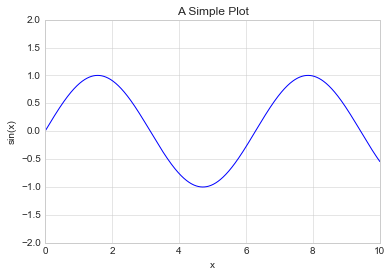This notebook contains an excerpt from the Python Data Science Handbook by Jake VanderPlas; the content is available on GitHub.

The text is released under the CC-BY-NC-ND license, and code is released under the MIT license. If you find this content useful, please consider supporting the work by buying the book!

# Simple Line Plots¶

Perhaps the simplest of all plots is the visualization of a single function $y = f(x)$. Here we will take a first look at creating a simple plot of this type. As with all the following sections, we'll start by setting up the notebook for plotting and importing the packages we will use:

In :
%matplotlib inline
import matplotlib.pyplot as plt
plt.style.use('seaborn-whitegrid')
import numpy as np


For all Matplotlib plots, we start by creating a figure and an axes. In their simplest form, a figure and axes can be created as follows:

In :
fig = plt.figure()
ax = plt.axes()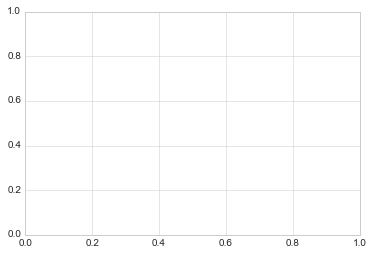In Matplotlib, the figure (an instance of the class plt.Figure) can be thought of as a single container that contains all the objects representing axes, graphics, text, and labels. The axes (an instance of the class plt.Axes) is what we see above: a bounding box with ticks and labels, which will eventually contain the plot elements that make up our visualization. Throughout this book, we'll commonly use the variable name fig to refer to a figure instance, and ax to refer to an axes instance or group of axes instances.

Once we have created an axes, we can use the ax.plot function to plot some data. Let's start with a simple sinusoid:

In :
fig = plt.figure()
ax = plt.axes()

x = np.linspace(0, 10, 1000)
ax.plot(x, np.sin(x));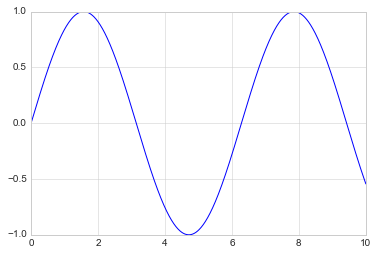Alternatively, we can use the pylab interface and let the figure and axes be created for us in the background (see Two Interfaces for the Price of One for a discussion of these two interfaces):

In :
plt.plot(x, np.sin(x));If we want to create a single figure with multiple lines, we can simply call the plot function multiple times:

In :
plt.plot(x, np.sin(x))
plt.plot(x, np.cos(x));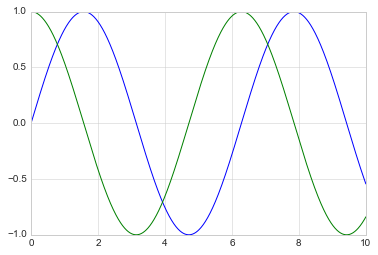That's all there is to plotting simple functions in Matplotlib! We'll now dive into some more details about how to control the appearance of the axes and lines.

## Adjusting the Plot: Line Colors and Styles¶

The first adjustment you might wish to make to a plot is to control the line colors and styles. The plt.plot() function takes additional arguments that can be used to specify these. To adjust the color, you can use the color keyword, which accepts a string argument representing virtually any imaginable color. The color can be specified in a variety of ways:

In :
plt.plot(x, np.sin(x - 0), color='blue')        # specify color by name
plt.plot(x, np.sin(x - 1), color='g')           # short color code (rgbcmyk)
plt.plot(x, np.sin(x - 2), color='0.75')        # Grayscale between 0 and 1
plt.plot(x, np.sin(x - 3), color='#FFDD44')     # Hex code (RRGGBB from 00 to FF)
plt.plot(x, np.sin(x - 4), color=(1.0,0.2,0.3)) # RGB tuple, values 0 to 1
plt.plot(x, np.sin(x - 5), color='chartreuse'); # all HTML color names supported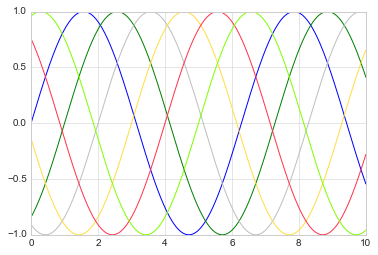If no color is specified, Matplotlib will automatically cycle through a set of default colors for multiple lines.

Similarly, the line style can be adjusted using the linestyle keyword:

In :
plt.plot(x, x + 0, linestyle='solid')
plt.plot(x, x + 1, linestyle='dashed')
plt.plot(x, x + 2, linestyle='dashdot')
plt.plot(x, x + 3, linestyle='dotted');

# For short, you can use the following codes:
plt.plot(x, x + 4, linestyle='-')  # solid
plt.plot(x, x + 5, linestyle='--') # dashed
plt.plot(x, x + 6, linestyle='-.') # dashdot
plt.plot(x, x + 7, linestyle=':');  # dotted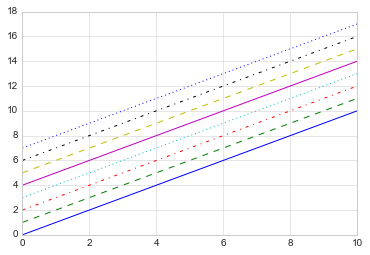If you would like to be extremely terse, these linestyle and color codes can be combined into a single non-keyword argument to the plt.plot() function:

In :
plt.plot(x, x + 0, '-g')  # solid green
plt.plot(x, x + 1, '--c') # dashed cyan
plt.plot(x, x + 2, '-.k') # dashdot black
plt.plot(x, x + 3, ':r');  # dotted red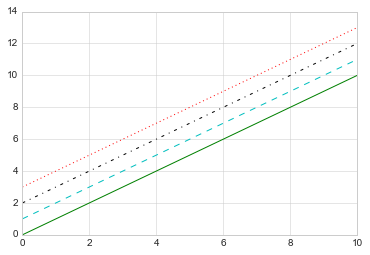These single-character color codes reflect the standard abbreviations in the RGB (Red/Green/Blue) and CMYK (Cyan/Magenta/Yellow/blacK) color systems, commonly used for digital color graphics.

There are many other keyword arguments that can be used to fine-tune the appearance of the plot; for more details, I'd suggest viewing the docstring of the plt.plot() function using IPython's help tools (See Help and Documentation in IPython).

## Adjusting the Plot: Axes Limits¶

Matplotlib does a decent job of choosing default axes limits for your plot, but sometimes it's nice to have finer control. The most basic way to adjust axis limits is to use the plt.xlim() and plt.ylim() methods:

In :
plt.plot(x, np.sin(x))

plt.xlim(-1, 11)
plt.ylim(-1.5, 1.5);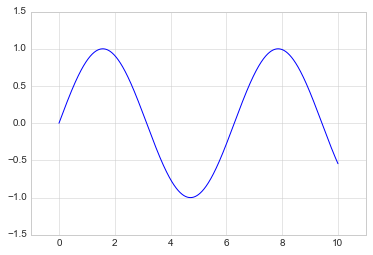If for some reason you'd like either axis to be displayed in reverse, you can simply reverse the order of the arguments:

In :
plt.plot(x, np.sin(x))

plt.xlim(10, 0)
plt.ylim(1.2, -1.2);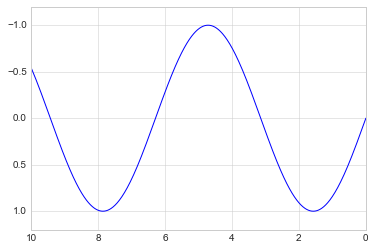A useful related method is plt.axis() (note here the potential confusion between axes with an e, and axis with an i). The plt.axis() method allows you to set the x and y limits with a single call, by passing a list which specifies [xmin, xmax, ymin, ymax]:

In :
plt.plot(x, np.sin(x))
plt.axis([-1, 11, -1.5, 1.5]);The plt.axis() method goes even beyond this, allowing you to do things like automatically tighten the bounds around the current plot:

In :
plt.plot(x, np.sin(x))
plt.axis('tight');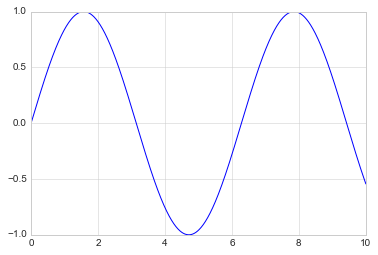It allows even higher-level specifications, such as ensuring an equal aspect ratio so that on your screen, one unit in x is equal to one unit in y:

In :
plt.plot(x, np.sin(x))
plt.axis('equal');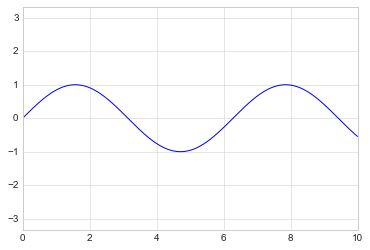For more information on axis limits and the other capabilities of the plt.axis method, refer to the plt.axis docstring.

## Labeling Plots¶

As the last piece of this section, we'll briefly look at the labeling of plots: titles, axis labels, and simple legends.

Titles and axis labels are the simplest such labels—there are methods that can be used to quickly set them:

In :
plt.plot(x, np.sin(x))
plt.title("A Sine Curve")
plt.xlabel("x")
plt.ylabel("sin(x)");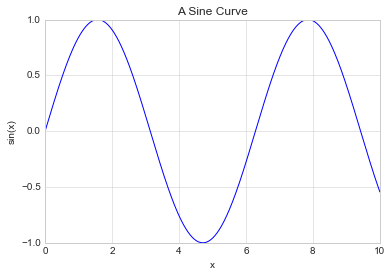The position, size, and style of these labels can be adjusted using optional arguments to the function. For more information, see the Matplotlib documentation and the docstrings of each of these functions.

When multiple lines are being shown within a single axes, it can be useful to create a plot legend that labels each line type. Again, Matplotlib has a built-in way of quickly creating such a legend. It is done via the (you guessed it) plt.legend() method. Though there are several valid ways of using this, I find it easiest to specify the label of each line using the label keyword of the plot function:

In :
plt.plot(x, np.sin(x), '-g', label='sin(x)')
plt.plot(x, np.cos(x), ':b', label='cos(x)')
plt.axis('equal')

plt.legend();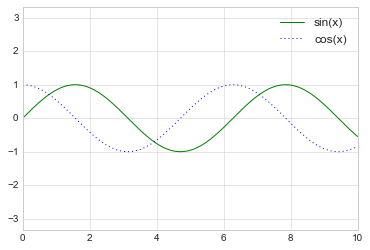As you can see, the plt.legend() function keeps track of the line style and color, and matches these with the correct label. More information on specifying and formatting plot legends can be found in the plt.legend docstring; additionally, we will cover some more advanced legend options in Customizing Plot Legends.

## Aside: Matplotlib Gotchas¶

While most plt functions translate directly to ax methods (such as plt.plot()ax.plot(), plt.legend()ax.legend(), etc.), this is not the case for all commands. In particular, functions to set limits, labels, and titles are slightly modified. For transitioning between MATLAB-style functions and object-oriented methods, make the following changes:

• plt.xlabel()ax.set_xlabel()
• plt.ylabel()ax.set_ylabel()
• plt.xlim()ax.set_xlim()
• plt.ylim()ax.set_ylim()
• plt.title()ax.set_title()

In the object-oriented interface to plotting, rather than calling these functions individually, it is often more convenient to use the ax.set() method to set all these properties at once:

In :
ax = plt.axes()
ax.plot(x, np.sin(x))
ax.set(xlim=(0, 10), ylim=(-2, 2),
xlabel='x', ylabel='sin(x)',
title='A Simple Plot');